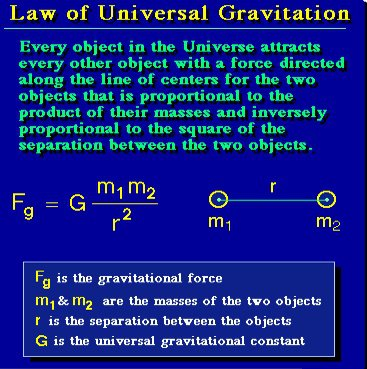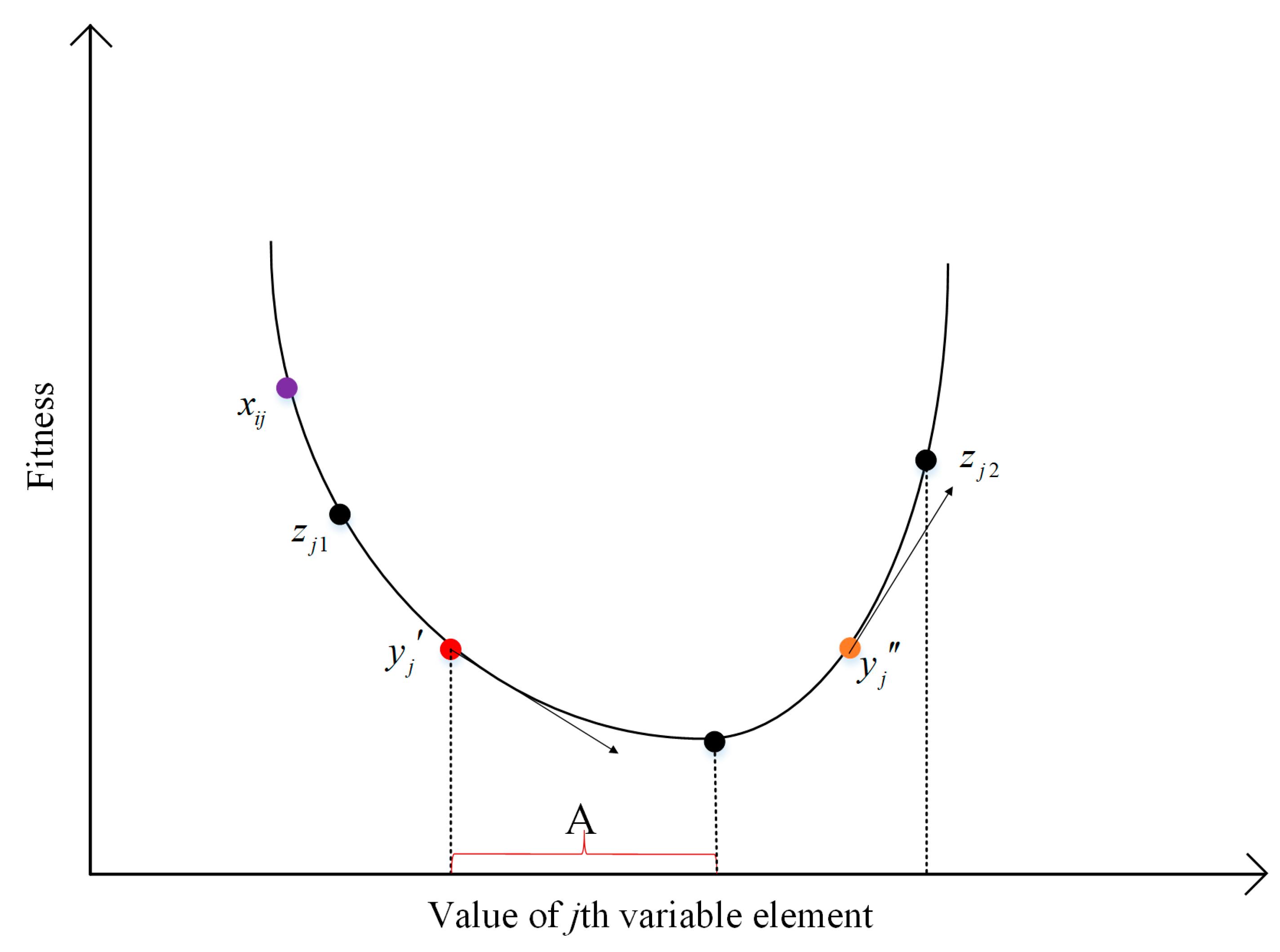# Acceleration Due to Gravity

• Astrophysics• Electricity• Electromagnetism• Energy• Fields• Force• Mechanics• Momentum• Nuclear Physics• Quantities & Units• States of Matter• Waves• Key ExperimentsGravity is the force that pulls objects towards the centre of the earth. This force is called the gravitational force, and it's what makes objects have weight. We use the letter g to represent the acceleration due to gravity. Basically, g tells us how fast an object will fall towards the earth.

According to Newton's second law of motion, the formula to calculate force is F = ma. We can replace a with g to get F = mg. This formula tells us the weight of an object under the influence of gravity. We use the unit N (Newton) or kg⋅m/s to measure weight. Sir Isaac Newton discovered this unit. The weight of an object depends on its location because it's affected by the force of gravity. For example, an object will weigh more at sea level than on top of a mountain, although the difference will be small. The weight of an object is a vector quantity because it has both magnitude and direction.

## Acceleration due to gravity on the surface of the earth

For a symmetrical object, the gravitational force acts towards the centre of the object. The value of g is almost constant near the surface of the earth, but as we move far from the surface of the earth, the strength of gravity decreases as the height increases.

The acceleration produced in any freely-falling body due to the force of gravity of another object, such as a planet, is known as acceleration due to gravity.An object with mass m under the influence of a bigger body, such as a planet with mass M

Scientists have found that the acceleration due to gravity decreases as the distance of an object from the centre of mass of a larger object increases. This distance, denoted by r, is measured from the centre of the earth. The acceleration due to gravity is inversely proportional to r^2 and directly proportional to the mass of the larger object, which is the earth in this case. This can be written as m‹‹M, where m is the mass of the object and M is the mass of the earth. Combining these two proportionalities gives us a formula for the acceleration due to gravity. The value of G, the constant of proportionality, for the earth is 6.674⋅10-11 Nm2 kg-2. If the object is at a height h above the surface of the earth, the formula changes to (§), which shows that as h increases, the force of gravity decreases.

## Acceleration due to gravity below the surface of the earth

The acceleration due to gravity does not follow the quadratic relationship when the object is below the surface of the earth. In fact, acceleration and distance are linearly dependent on each other for r < R (below the surface of the earth).

If an object is at r distance from the centre of the earth, the mass of the earth responsible for the acceleration due to gravity at that point will be:

This can be easily deduced using the formula for the volume of a sphere.

We have assumed the earth to be a sphere, but in reality, the radius of the earth is at its minimum at the poles and at its maximum at the equator. The difference is quite small, and so we assume the earth to be a sphere for simplified calculations. The acceleration due to gravity follows the proportionality explained earlier:

Substituting for m, we get:

We can now see that as G, M, and R are constants for a given object or planet, the acceleration linearly depends on r. Hence, we see that as r approaches R, the acceleration due to gravity increases according to the above linear relation, after which it decreases according to §, which we derived earlier. In practice, most of the real-world problems include the object being outside the surface of the earth.

## Geometric interpretation of acceleration due to gravityThe graph of g as a function of r, which is linear until r = R and has a parabolic curve for r > R

## ‍

The acceleration due to gravity has a linear relationship with distance until the surface of the earth. After that, it follows a quadratic relationship. This is illustrated by a parabolic graph. The weight of an object is calculated using the formula F=mg, where g is the acceleration due to gravity. To calculate g at a different distance from the earth's surface, we use the equation g=G*M/(R+h)^2, where G is the gravitational constant, M is the mass of the earth, R is the radius of the earth, and h is the distance from the surface of the earth. It's important to convert all units to SI units and specify the units of the calculated quantity. In an example problem, we can use this equation to calculate the weight of an object in orbit based on its weight on the surface of the earth. The acceleration due to gravity is always towards the centre of mass of the larger object and decreases as we move farther away from the surface of the earth.

## Acceleration Due to Gravity

Does mass affect acceleration due to gravity?

Acceleration due to gravity is not affected by the mass of the object itself, but it is affected by the mass of the body or planet it is attracted to.

What is acceleration due to gravity?

The acceleration produced in any freely-falling body due to the force of gravity of another object, such as a planet, is known as acceleration due to gravity.

What opposes acceleration due to gravity?

When there is no external force being applied to the object, the only force that opposes acceleration due to gravity is air resistance.

Can the acceleration due to gravity be negative?

Conventionally, the Cartesian y-axis is taken as negative towards the downward direction, and as acceleration due to gravity acts downwards, it is negative.

Does acceleration due to gravity change with latitude?

The earth is not a perfect sphere, with its radius decreasing as we go from the equator to the poles, and so acceleration due to gravity changes with latitude. Having said that, the change in magnitude is quite small.14-day free trial. Cancel anytime.Join 10,000+ learners worldwide.The first 14 days are on us96% of learners report x2 faster learningFree hands-on onboarding & supportCancel Anytime